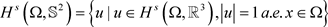# 一类分数阶调和映射迭代算法的收敛性Convergence of Iterative Algorithm for a Class of Fractional Harmonic Maps

DOI: 10.12677/AAM.2021.104139, PDF, HTML, XML, 下载: 10  浏览: 38

Abstract: This paper proposes an iterative algorithm for fractional harmonic maps and its convergence is proved.

1. 引言

$\Omega$${ℝ}^{N}$ 中的光滑有界区域， $N=1,2,3$${\mathbb{S}}^{2}$${ℝ}^{\text{3}}$ 中的标准单位球， $\text{0}。定义映射 $u:\Omega \to {\mathbb{S}}^{2}$ 的非局部能量为

${E}_{s}\left(u\right)=\frac{1}{2}{\iint }_{\Omega ×\Omega }\frac{{|u\left(x\right)-u\left(y\right)|}^{2}}{{|x-y|}^{n+2s}}\text{d}x\text{d}y,$ (1)

${H}^{s}\left(\Omega ,{ℝ}^{3}\right):=\left\{u:\Omega \to {ℝ}^{3}|{E}_{s}\left(u\right)+{\int }_{\Omega }{|u|}^{2}\text{d}x<\infty \right\}$

${{E}^{\prime }}_{s}\left(u\right)\left[v\right]={\iint }_{\Omega ×\Omega }\frac{\left(u\left(x\right)-u\left(y\right)\right)\cdot \left(v\left(x\right)-v\left(y\right)\right)}{{|x-y|}^{n+2s}}\text{d}x\text{d}y.$${H}_{0}^{s}\left(\Omega ,{ℝ}^{3}\right)=\left\{u|u\in {H}^{s}\left(\Omega ,{ℝ}^{3}\right),{u|}_{\partial \Omega }=\stackrel{\to }{0}\right\}.$(2)

$u\in {\stackrel{˙}{H}}^{s}\left(\Omega ,\mathcal{N}\right)$，其中， $\mathcal{N}\subset {ℝ}^{m}$ 是无边的k-维光滑子流形。如果函数u是泛函 ${E}_{s}\left(u\right)$ 在扰动 $t↦{\Pi }_{\mathcal{N}}\left(u+t\phi \right)$ 下的临界点，则称u为s-阶调和映射。其中， $\phi \in {C}_{c}^{\infty }\left(\Omega \right)$${\Pi }_{\mathcal{N}}:{ℝ}^{m}\to \mathcal{N}$${ℝ}^{m}$ 到子流形 $\mathcal{N}$ 上的投影映射。当s = 1时，这是经典调和映射的概念，文献   和  对调和映射的当前研究情况进行了系统的总结。近些年以来，人们对s为非整数的情形非常感兴趣，比如当靶流形 $\mathcal{N}$ 为标准黎曼球面时，文献  利用三交换子估计方法，证明了1/2-阶调和映射的光滑性；文献  得到了n/2-阶调和映射的光滑性；文献  分析了1/2-阶调和映射的紧致性和爆破性质；文献  证明了n/2-阶调和映射的Hölder连续性。当靶流形 $\mathcal{N}$ 为一般的紧致黎曼流形时，文献  得到了1/2-阶调和映射的Hölder连续性。文献  证明了靶流形分别为球和黎曼齐次流形时积分微分调和映射热流整体弱解的存在性。Alouges在文献  给出了一类经典调和映射能量极小问题的迭代算法，文献  讨论调和映射逼近格式的稳定性和收敛性，介绍了一种基于Alouges的迭代算法的有限元离散化方法，并证明了它仅对锐角型三角剖分是稳定和收敛的，并提出了一个后验准则，使我们能够得到一般三角剖分上调和映射弱收敛的充分条件和自适应网格细化的充分条件，数值模拟实验进一步表明，自适应策略可以自动细化典型奇异点附近的三角剖分，从而提高其运算效率。

$\underset{w\in {K}_{u}}{\mathrm{min}}{\int }_{\Omega }{\int }_{\Omega }\frac{{|{u}_{n}\left(x\right)-w\left(x\right)-{u}_{n}\left(y\right)+w\left(y\right)|}^{2}}{{|x-y|}^{n+2s}}\text{d}x\text{d}y.$ (3)

${w}_{n}=w\left({u}_{n}\right)$ 为此问题的解。第二步，令 ${u}_{n+1}:=\frac{{u}_{n}-{w}_{n}}{|{u}_{n}-{w}_{n}|}$

$0={\int }_{\Omega }{\int }_{\Omega }\frac{\left(u\left(x\right)-u\left(y\right)\right)\cdot \left(\psi \left(x\right)-\psi \left(y\right)\right)}{{|x-y|}^{n+2s}}\text{d}x\text{d}y,\text{ }\forall \psi \in {K}_{u}.$

2. 定理1的证明

$\begin{array}{l}{\int }_{\Omega }{\int }_{\Omega }\frac{{|{u}_{n}\left(x\right)-{u}_{n}\left(y\right)|}^{2}}{{|x-y|}^{n+2s}}\text{d}x\text{d}y\\ ={\int }_{\Omega }{\int }_{\Omega }\frac{{|{u}_{n}\left(x\right)-{w}_{n}\left(x\right)-{u}_{n}\left(y\right)+{w}_{n}\left(y\right)|}^{2}}{{|x-y|}^{n+2s}}\text{d}x\text{d}y+{\int }_{\Omega }{\int }_{\Omega }\frac{{|{w}_{n}\left(x\right)-{w}_{n}\left(y\right)|}^{2}}{{|x-y|}^{n+2s}}\text{d}x\text{d}y.\end{array}$

${\int }_{\Omega }{\int }_{\Omega }\frac{\left({u}_{n}\left(x\right)-w\left(x\right)-{u}_{n}\left(y\right)+w\left(y\right)\right)\left(\psi \left(x\right)-\psi \left(y\right)\right)}{{|x-y|}^{n+2s}}\text{d}x\text{d}y=0.$

$\psi ={w}_{n}$，我们得到

$\begin{array}{l}{\int }_{\Omega }{\int }_{\Omega }\frac{{|{u}_{n}\left(x\right)-{u}_{n}\left(y\right)|}^{2}}{{|x-y|}^{n+2s}}\text{d}x\text{d}y\\ ={\int }_{\Omega }{\int }_{\Omega }\frac{{|{u}_{n}\left(x\right)-{w}_{n}\left(x\right)-{u}_{n}\left(y\right)+{w}_{n}\left(y\right)|}^{2}}{{|x-y|}^{n+2s}}\text{d}x\text{d}y+{\int }_{\Omega }{\int }_{\Omega }\frac{{|{w}_{n}\left(x\right)-{w}_{n}\left(y\right)|}^{2}}{{|x-y|}^{n+2s}}\text{d}x\text{d}y\\ \text{\hspace{0.17em}}\text{\hspace{0.17em}}+2{\int }_{\Omega }{\int }_{\Omega }\frac{\left({u}_{n}\left(x\right)-w\left(x\right)-{u}_{n}\left(y\right)+w\left(y\right)\right)\left(\psi \left(x\right)-\psi \left(y\right)\right)}{{|x-y|}^{n+2s}}\text{d}x\text{d}y\\ ={\int }_{\Omega }{\int }_{\Omega }\frac{{|{u}_{n}\left(x\right)-{w}_{n}\left(x\right)-{u}_{n}\left(y\right)+{w}_{n}\left(y\right)|}^{2}}{{|x-y|}^{n+2s}}\text{d}x\text{d}y+{\int }_{\Omega }{\int }_{\Omega }\frac{{|{w}_{n}\left(x\right)-{w}_{n}\left(y\right)|}^{2}}{{|x-y|}^{n+2s}}\text{d}x\text{d}y.\end{array}$

${\int }_{\Omega }{\int }_{\Omega }\frac{{|{u}_{n+1}\left(x\right)-{u}_{n+1}\left(y\right)|}^{2}}{{|x-y|}^{n+2s}}\text{d}x\text{d}y\le {\int }_{\Omega }{\int }_{\Omega }\frac{{|{u}_{n}\left(x\right)-{w}_{n}\left(x\right)-{u}_{n}\left(y\right)+{w}_{n}\left(y\right)|}^{2}}{{|x-y|}^{n+2s}}\text{d}x\text{d}y.$

$\begin{array}{l}{\int }_{\Omega }{\int }_{\Omega }\frac{{|{u}_{n+1}\left(x\right)-{u}_{n+1}\left(y\right)|}^{2}}{{|x-y|}^{n+2s}}\text{d}x\text{d}y={\int }_{\Omega }{\int }_{\Omega }\frac{{|\frac{{u}_{n}-{w}_{n}}{|{u}_{n}-{w}_{n}|}\left(x\right)-\frac{{u}_{n}-{w}_{n}}{|{u}_{n}-{w}_{n}|}\left(y\right)|}^{2}}{{|x-y|}^{n+2s}}\text{d}x\text{d}y\\ ={\int }_{\Omega }{\int }_{\Omega }\frac{2-2\frac{\left({u}_{n}\left(x\right)-{w}_{n}\left(x\right)\right)\cdot \left({u}_{n}\left(y\right)-{w}_{n}\left(y\right)\right)}{|{u}_{n}\left(x\right)-{w}_{n}\left(x\right)||{u}_{n}\left(y\right)-{w}_{n}\left(y\right)|}}{{|x-y|}^{n+2s}}\text{d}x\text{d}y\\ \le {\int }_{\Omega }{\int }_{\Omega }\frac{2|{u}_{n}\left(x\right)-{w}_{n}\left(x\right)||{u}_{n}\left(y\right)-{w}_{n}\left(y\right)|-2\left({u}_{n}\left(x\right)-{w}_{n}\left(x\right)\right)\cdot \left({u}_{n}\left(y\right)-{w}_{n}\left(y\right)\right)}{{|x-y|}^{n+2s}}\text{d}x\text{d}y\\ \le {\int }_{\Omega }{\int }_{\Omega }\frac{{|{u}_{n}\left(x\right)-{w}_{n}\left(x\right)-{u}_{n}\left(y\right)+{w}_{n}\left(y\right)|}^{2}}{{|x-y|}^{n+2s}}\text{d}x\text{d}y.\end{array}$

$\begin{array}{c}{\int }_{\Omega }{\int }_{\Omega }\frac{{|{u}_{n+1}\left(x\right)-{u}_{n+1}\left(y\right)|}^{2}}{{|x-y|}^{n+2s}}\text{d}x\text{d}y\le {\int }_{\Omega }{\int }_{\Omega }\frac{{|{u}_{n}\left(x\right)-{w}_{n}\left(x\right)-{u}_{n}\left(y\right)+{w}_{n}\left(y\right)|}^{2}}{{|x-y|}^{n+2s}}\text{d}x\text{d}y\\ ={\int }_{\Omega }{\int }_{\Omega }\frac{{|{u}_{n}\left(x\right)-{u}_{n}\left(y\right)|}^{2}}{{|x-y|}^{n+2s}}\text{d}x\text{d}y-{\int }_{\Omega }{\int }_{\Omega }\frac{{|{w}_{n}\left(x\right)-{w}_{n}\left(y\right)|}^{2}}{{|x-y|}^{n+2s}}\text{d}x\text{d}y.\end{array}$

${\int }_{\Omega }{\int }_{\Omega }\frac{{|{w}_{n}\left(x\right)-{w}_{n}\left(y\right)|}^{2}}{{|x-y|}^{n+2s}}\text{d}x\text{d}y\le {\int }_{\Omega }{\int }_{\Omega }\frac{{|{u}_{n}\left(x\right)-{u}_{n}\left(y\right)|}^{2}}{{|x-y|}^{n+2s}}\text{d}x\text{d}y-{\int }_{\Omega }{\int }_{\Omega }\frac{{|{u}_{n+1}\left(x\right)-{u}_{n+1}\left(y\right)|}^{2}}{{|x-y|}^{n+2s}}\text{d}x\text{d}y.$

$\underset{n=0}{\overset{N}{\sum }}{\int }_{\Omega }{\int }_{\Omega }\frac{{|{w}_{n}\left(x\right)-{w}_{n}\left(y\right)|}^{2}}{{|x-y|}^{n+2s}}\text{d}x\text{d}y\le {\int }_{\Omega }{\int }_{\Omega }\frac{{|{u}_{0}\left(x\right)-{u}_{0}\left(y\right)|}^{2}}{{|x-y|}^{n+2s}}\text{d}x\text{d}y.$

${\int }_{\Omega }{\int }_{\Omega }\frac{\left({u}_{n}\left(x\right)-{w}_{n}\left(x\right)-{u}_{n}\left(y\right)+w\left(y\right)\right)\left(\Psi \left(x\right)-\Psi \left(y\right)\right)}{{|x-y|}^{n+2s}}\text{d}x\text{d}y=0,\text{ }\Psi \in {K}_{{u}_{n}}.$

$\Psi =\varphi ×{u}_{n}$，这里 $\varphi \in {C}_{0}^{\infty }\left(\Omega ,{ℝ}^{3}\right)$，于是我们有

${\int }_{\Omega }{\int }_{\Omega }\frac{\left({u}_{n}\left(x\right)-{w}_{n}\left(x\right)-{u}_{n}\left(y\right)+{w}_{n}\left(y\right)\right)\left(\varphi \left(x\right)×{u}_{n}\left(x\right)-\varphi \left(y\right)×{u}_{n}\left(y\right)\right)}{{|x-y|}^{n+2s}}\text{d}x\text{d}y=0.$

$\begin{array}{c}0={\int }_{\Omega }{\int }_{\Omega }\frac{\left({u}_{n}\left(x\right)-{w}_{n}\left(x\right)-{u}_{n}\left(y\right)+{w}_{n}\left(y\right)\right)\left(\varphi \left(x\right)×{u}_{n}\left(x\right)-\varphi \left(y\right)×{u}_{n}\left(y\right)\right)}{{|x-y|}^{n+2s}}\text{d}x\text{d}y\\ ={\int }_{\Omega }{\int }_{\Omega }\frac{\left({u}_{n}\left(x\right)-{w}_{n}\left(x\right)-{u}_{n}\left(y\right)+{w}_{n}\left(y\right)\right)\left(\varphi \left(x\right)-\varphi \left(y\right)\right)×{u}_{n}\left(x\right)}{{|x-y|}^{n+2s}}\text{d}x\text{d}y\\ \text{\hspace{0.17em}}\text{\hspace{0.17em}}+{\int }_{\Omega }{\int }_{\Omega }\frac{\left({u}_{n}\left(x\right)-{w}_{n}\left(x\right)-{u}_{n}\left(y\right)+{w}_{n}\left(y\right)\right)\left[\varphi \left(y\right)×\left({u}_{n}\left(x\right)-{u}_{n}\left(y\right)\right)\right]}{{|x-y|}^{n+2s}}\text{d}x\text{d}y\\ ={\int }_{\Omega }{\int }_{\Omega }\frac{\left({u}_{n}\left(x\right)-{u}_{n}\left(y\right)\right)\left(\varphi \left(x\right)-\varphi \left(y\right)\right)×{u}_{n}\left(x\right)}{{|x-y|}^{n+2s}}\text{d}x\text{d}y\\ \text{\hspace{0.17em}}\text{\hspace{0.17em}}-{\int }_{\Omega }{\int }_{\Omega }\frac{\left({w}_{n}\left(x\right)-{w}_{n}\left(y\right)\right)\left(\varphi \left(x\right)-\varphi \left(y\right)\right)×{u}_{n}\left(x\right)}{{|x-y|}^{n+2s}}\text{d}x\text{d}y\end{array}$

$\begin{array}{l}\text{\hspace{0.17em}}\text{\hspace{0.17em}}-{\int }_{\Omega }{\int }_{\Omega }\frac{\left({w}_{n}\left(x\right)-{w}_{n}\left(y\right)\right)\left[\varphi \left(y\right)×\left({u}_{n}\left(x\right)-{u}_{n}\left(y\right)\right)\right]}{{|x-y|}^{n+2s}}\text{d}x\text{d}y\\ ={\int }_{\Omega }{\int }_{\Omega }\frac{\left(\varphi \left(x\right)-\varphi \left(y\right)\right)\cdot \left[{u}_{n}\left(x\right)×\left({u}_{n}\left(x\right)-{u}_{n}\left(y\right)\right)\right]}{{|x-y|}^{n+2s}}\text{d}x\text{d}y\\ \text{\hspace{0.17em}}\text{\hspace{0.17em}}-{\int }_{\Omega }{\int }_{\Omega }\frac{\left(\varphi \left(x\right)-\varphi \left(y\right)\right)\cdot \left[{u}_{n}\left(x\right)×\left({w}_{n}\left(x\right)-{w}_{n}\left(y\right)\right)\right]}{{|x-y|}^{n+2s}}\text{d}x\text{d}y\\ \text{\hspace{0.17em}}\text{\hspace{0.17em}}-{\int }_{\Omega }{\int }_{\Omega }\frac{\varphi \left(y\right)\cdot \left[\left({u}_{n}\left(x\right)-{u}_{n}\left(y\right)\right)×\left({w}_{n}\left(x\right)-{w}_{n}\left(y\right)\right)\right]}{{|x-y|}^{n+2s}}\text{d}x\text{d}y,\end{array}$

$0={\int }_{\Omega }{\int }_{\Omega }\frac{\left(\varphi \left(x\right)-\varphi \left(y\right)\right)\cdot \left[{u}_{\infty }\left(x\right)×\left({u}_{\infty }\left(x\right)-{u}_{\infty }\left(y\right)\right)\right]}{{|x-y|}^{n+2s}}\text{d}x\text{d}y.$

$0={\int }_{\Omega }{\int }_{\Omega }\frac{\left({u}_{\infty }\left(x\right)-{u}_{\infty }\left(y\right)\right)\cdot \left[{u}_{\infty }\left(x\right)×\varphi \left(x\right)-{u}_{\infty }\left(y\right)×\varphi \left(y\right)\right]}{{|x-y|}^{n+2s}}\text{d}x\text{d}y.$

$\begin{array}{c}{u}_{\infty }\left(x\right)×\varphi \left(x\right)={u}_{\infty }\left(x\right)×\left({u}_{\infty }\left(x\right)×\psi \left(x\right)\right)\\ =\left({u}_{\infty }\left(x\right)\cdot \psi \left(x\right)\right){u}_{\infty }\left(x\right)-\left({u}_{\infty }\left(x\right)\cdot {u}_{\infty }\left(x\right)\right)\psi \left(x\right)\\ =\psi \left(x\right),\end{array}$

$0={\int }_{\Omega }{\int }_{\Omega }\frac{\left({u}_{\infty }\left(x\right)-{u}_{\infty }\left(y\right)\right)\cdot \left(\psi \left(x\right)-\psi \left(y\right)\right)}{{|x-y|}^{n+2s}}\text{d}x\text{d}y.$

${\int }_{\Omega }{\int }_{\Omega }\frac{\left(u\left(x\right)-{w}_{1}\left(x\right)-u\left(y\right)+{w}_{1}\left(y\right)\right)\cdot \left({w}_{1}\left(x\right)-{w}_{2}\left(x\right)-{w}_{1}\left(y\right)+{w}_{2}\left(y\right)\right)}{{|x-y|}^{n+2s}}\text{d}x\text{d}y$

${\int }_{\Omega }{\int }_{\Omega }\frac{\left(u\left(x\right)-{w}_{2}\left(x\right)-u\left(y\right)+{w}_{2}\left(y\right)\right)\cdot \left({w}_{1}\left(x\right)-{w}_{2}\left(x\right)-{w}_{1}\left(y\right)+{w}_{2}\left(y\right)\right)}{{|x-y|}^{n+2s}}\text{d}x\text{d}y$

${\int }_{\Omega }{\int }_{\Omega }\frac{\left(u\left(x\right)-u\left(y\right)\right)\cdot \left(\Psi \left(x\right)-\Psi \left(y\right)\right)}{{|x-y|}^{n+2s}}\text{d}x\text{d}y=0,\text{ }\Psi \in {K}_{u}.$

  Helein, F. (1990) Regularite des applications faiblement harmoniques entre une surface et une sphere. Comptes Rendus de l’Académie des Sciences—Series I—Mathematics, 311, 519-524.  Lin, F. and Wang, C. (2008) The Analysis of Harmonic Maps and Their Heat Flows. World Scientific Publishing Co. Pte. Ltd., Hackensack, HJ. https://doi.org/10.1142/6679  Riviere, T. (2007) Conservation Laws for Conformally Invariant Variational Problems. Inventiones Mathematicae, 168, 1-22. https://doi.org/10.1007/s00222-006-0023-0  Da Lio, F. and Riviere, T. (2011) Three-Term Commutator Estimates and the Regularity of 1/2-Harmonic Maps into Spheres. Analysis & PDE, 4, 149-190. https://doi.org/10.2140/apde.2011.4.149  Da Lio, F. (2013) Fractional Harmonic Maps into Manifolds in Odd Dimension n > 1. Calculus of Variations and Partial Differential Equations, 48, 421-445. https://doi.org/10.1007/s00526-012-0556-6  Da Lio, F. (2015) Compactness and Bubble Analysis for 1/2-Harmonic Maps. Annales de l’Institut Henri Poincaré, 32, 201-224. https://doi.org/10.1016/j.anihpc.2013.11.003  Schikorra, A. (2012) Regularity of n/2-Harmonic Maps into Spheres. Journal of Differential Equations, 252, 1862-1911. https://doi.org/10.1016/j.jde.2011.08.021  Da Lio, F. and Riviere, T. (2011) Sub-Criticality of Non-Local Schrodinger Systems with Antisymmetric Potentials and Applications to Half-Harmonic Maps. Advances in Mathematics, 227, 1300-1348. https://doi.org/10.1016/j.aim.2011.03.011  Schikorra, A., Sire, Y. and Wang, C. (2017) Weak Solutions of Geometric Flows Associated to Integro-Differential Harmonic Maps. Manuscripta Mathematica, 153, 389-402. https://doi.org/10.1007/s00229-016-0899-y  Alouges, F. (1997) A New Algorithm for Computing Liquid Crystal Stable Configurations: The Harmonic Mapping Case. SIAM Journal on Numerical Analysis, 34, 1708-1726. https://doi.org/10.1137/S0036142994264249  Bartels, S. (2005) Stability and Convergence of Finite-Element Approximation Schemes for Harmonic Maps. SIAM Journal on Numerical Analysis, 43, 220-238. https://doi.org/10.1137/040606594